# RD Sharma Class 8 Solutions Chapter 1 Rational Numbers Ex 1.4

## RD Sharma Class 8 Solutions Chapter 1 Rational Numbers Ex 1.4

These Solutions are part of RD Sharma Class 8 Solutions. Here we have given RD Sharma Class 8 Solutions Chapter 1 Rational Numbers Ex 1.4

Other Exercises

Question 1.
Simplify each of the following and write as a rational number of the form :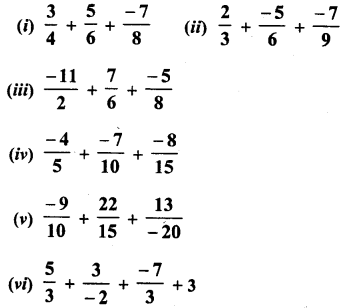Solution: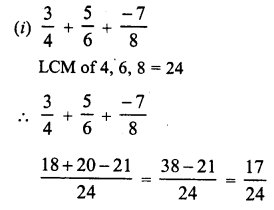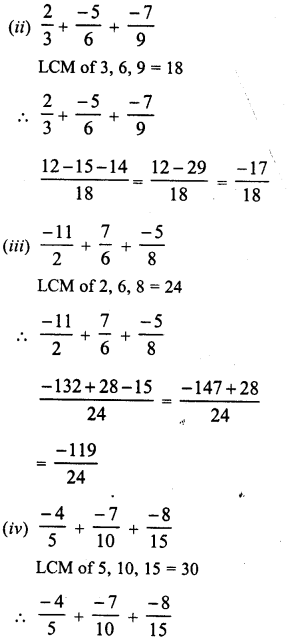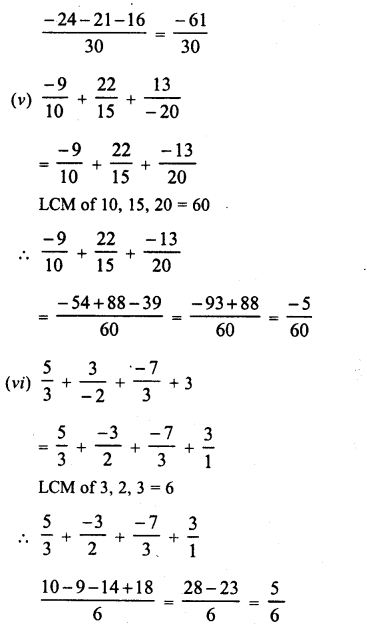Question 2.
Express each of the following as a rational number of the form $$\frac { p }{ q }$$: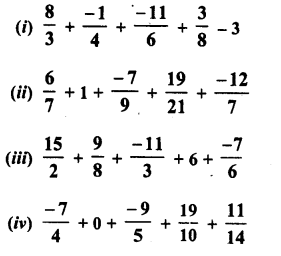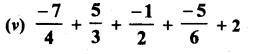Solution: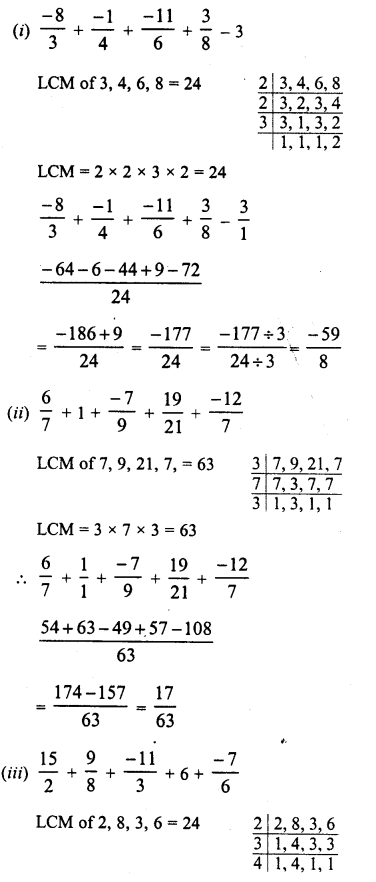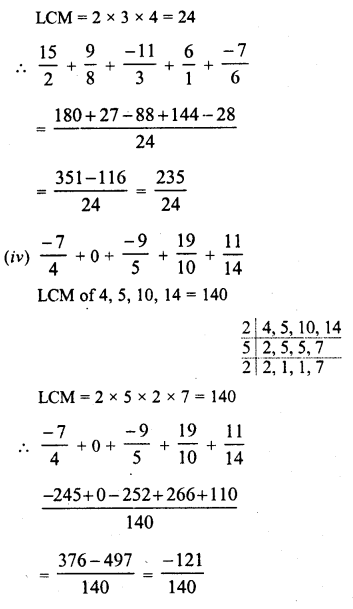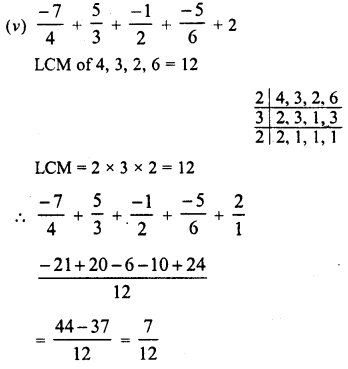Question 3.
Simplify :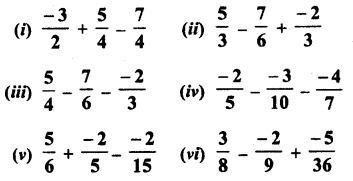Solution: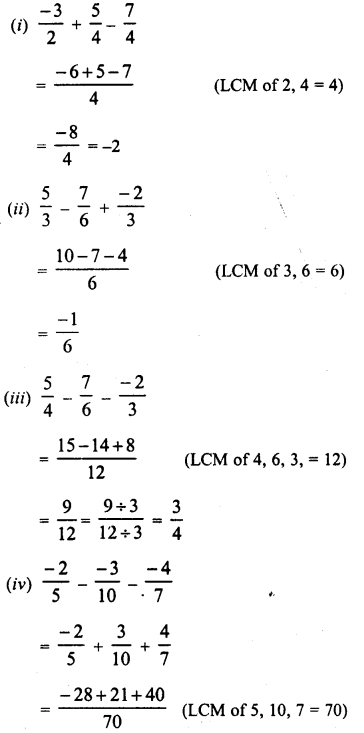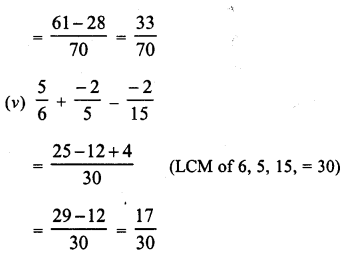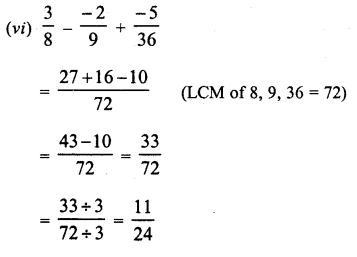Hope given RD Sharma Class 8 Solutions Chapter 1 Rational Numbers Ex 1.4 are helpful to complete your math homework.

If you have any doubts, please comment below. Learn Insta try to provide online math tutoring for you.